Question

# The mean starting salary for college graduates in 2016 was \$36,000. Assume that the distribution of...

The mean starting salary for college graduates in 2016 was \$36,000. Assume that the distribution of starting salaries follows the normal distribution with a standard deviation of \$3,000.

1.What is the probability that a graduate will have a starting salary more than \$43,500?

2. What is the probability that a graduate will have a starting salary between \$42,000 and \$43,500?

3. What is the probability that a graduate will have a starting salary between \$30,000 and \$45,000?

4. What starting salary (or more) will the top 3% of the graduates have?

5. 99.97% of the observations will lie between what values?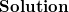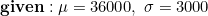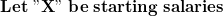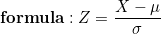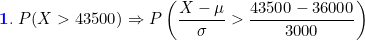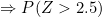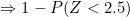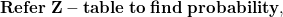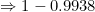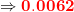The probability that a graduate will have a starting salary more than \$43,500 is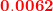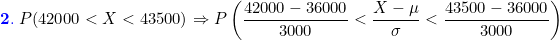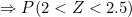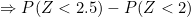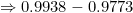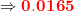The probability that a graduate will have a starting salary between \$42,000 and \$43,500 is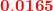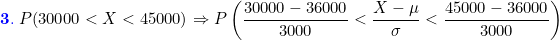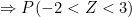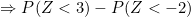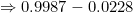The probability that a graduate will have a starting salary between \$30,000 and \$45,000 is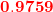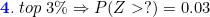From Z-table, Lookup for Z-value corresponding to area 0.03 to the right of the normal curve.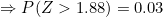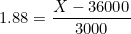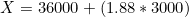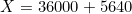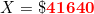The starting salary (or more) will the top 3% of the graduates have is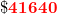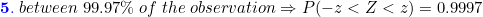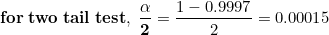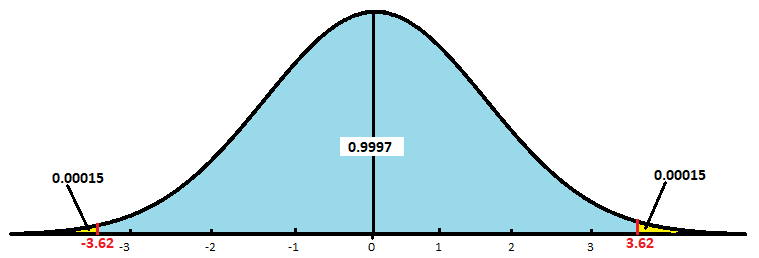From Z-table, Lookup for Z-value corresponding to area 0.00015 to the left of the normal curve.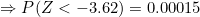From Z-table, Lookup for Z-value corresponding to area 0.00015 to the right of the normal curve.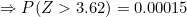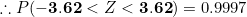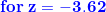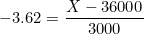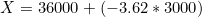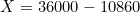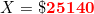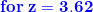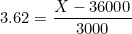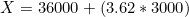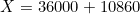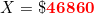The 99.97% of the observations will lie between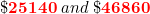#### Earn Coins

Coins can be redeemed for fabulous gifts.

Similar Homework Help Questions
• ### A survey reported that the mean starting salary for college graduates after a three-year program was...

A survey reported that the mean starting salary for college graduates after a three-year program was \$33,160.Assume that the distribution of starting salaries follows the normal distribution with a standard deviation of \$4090. What percentage of the graduates have starting salaries: (Round z-score computation to 2 decimal places and the final answers to 4 decimal places.)   a. Between \$32,500 and \$38,600? Probability            b. More than \$42,900? Probability            c. Between \$38,600 and \$42,900? Probability

• ### According to a National Association of Colleges and Employers, the average starting salary for new college...

According to a National Association of Colleges and Employers, the average starting salary for new college graduates in health sciences is \$51,541. The average starting salary for new college graduates in business is \$53,901.† Assume that starting salaries are normally distributed and that the standard deviation for starting salaries for new college graduates in health sciences is \$13,000. Assume that the standard deviation for starting salaries for new college graduates in business is \$17,000. What is the probability that a...

• ### According to the National Association of Colleges and Employers, the 2015 man starting salary for new...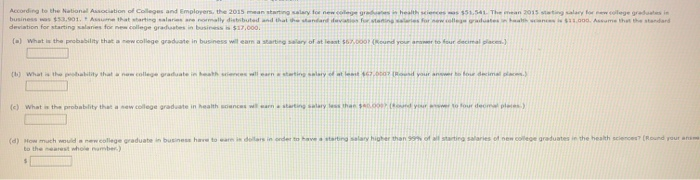According to the National Association of Colleges and Employers, the 2015 man starting salary for new college - \$51.541. The man 2015 stating for new colega grein business was \$33,001. Asume that starting sales normally distributed and that the des \$11.000. Assume that the standard deviation for starting lanes for new college graduates in business \$17,000. c) What the probability that a new college graduate in business wil eam a starting salary of at least 567.000 (Round your name to...

• ### The average starting salary of this year’s graduates of a large university (LU) is \$55,000 with...

The average starting salary of this year’s graduates of a large university (LU) is \$55,000 with a standard deviation of \$4,000. Furthermore, it is known that the starting salaries are normally distributed. What is the probability that a randomly selected LU graduate will have a starting salary of at least \$52,700? Individuals with starting salaries of less than \$45,00 receive a free class. What percentage of the graduates will receive the free class? What percent of graduates will have their...

• ### A study on salaries of recent graduates from a certain college are normally distributed with mean...

A study on salaries of recent graduates from a certain college are normally distributed with mean and standard deviation . Use the information to answer questions #1-6. You may use manual, technology, or table for computation, but you need to show work to justify your computation. 1. Suppose your starting salary is \$55,000. a. determine the z-score b. interpret your z-score in terms of percentile (ranking) in the context of the population. 2. Find proportion for which the salary of...

• ### Starting salaries of 64 college graduates who have taken a statistics course have a mean of...

Starting salaries of 64 college graduates who have taken a statistics course have a mean of \$43,500 with a standard deviation of \$6,800. Find a 68% confidence interval for μ.

• ### 2. The average starting salary for this year's graduates at a large university (LU) is \$50,000 wi...

2. The average starting salary for this year's graduates at a large university (LU) is \$50,000 with a standard deviation of \$8,000. Furthermore, it is known that the starting salaries are normally distributed. a. What is the probability that a randomly selected LU graduate will have a starting salary of at least \$61,900? b. Individuals with starting salaries of less than \$36,800 receive a low income tax break. What percentage of the graduates will receive the tax break? Please if...

• ### The average starting salary for an MBA graduate in the class of 2018 was ​\$112,000. Assume...

The average starting salary for an MBA graduate in the class of 2018 was ​\$112,000. Assume the population standard deviation for starting MBA salaries was ​\$19,000. A random sample of 30 MBA graduates from the class of 2018 was selected. a. What is the probability that the sample mean is less than ​\$119,000? b. What is the probability that the sample mean is more than ​\$105,000​? c. What is the probability that the sample mean is between ​\$112,000 and ​\$119,000​?...

• ### The average starting salary for an MBA graduate in the class of 2018 was \$102,000. Assume...

The average starting salary for an MBA graduate in the class of 2018 was \$102,000. Assume the population standard deviation for starting MBA salaries was ​\$16,000. A random sample of 35 MBA graduates from the class of 2018 was selected. a.What is the probability that the sample mean is less than \$104,000​? b. What is the probability that the sample mean is more than \$100,000​? c. What is the probability that the sample mean is between ​\$102,000 and \$104,000​? d....

• ### Suppose that the salary range for recent college graduates with a bachelor's degree in economics is...

Suppose that the salary range for recent college graduates with a bachelor's degree in economics is \$30,000 to \$50,000, with 25 percent of jobs offering \$30,000 per year, 50 percent offering \$40,000 per year and 25 percent offering \$50,000 per year and that in all other respects, the jobs are equally satisfying. Assume that in this market, a job offer remains open for only a short time so that continuing to search requires an applicant to reject any current job...